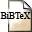# Upper-Bounding Rate Distortion Functions based on the Minimum Mean-Square Error

## Authors

M. Dörpinghaus, M. Rüngeler, R. Mathar,

## Abstract

We derive a new upper bound on the rate distortion function for arbitrary memoryless sources, which is based on the relation between mutual information and minimum mean-square error discovered by Guo et al. This upper bound is in general tighter than the well known upper bound given by the rate distortion function of a Gaussian source with an equal variance found by Shannon and becomes tight for Gaussian sources. We evaluate the new upper bound for various source distributions and compare it to the Shannon lower and upper bound and to the rate distortion function calculated with the Blahut Arimoto algorithm. This shows that the new upper bound is quite tight.

A Matlab tool to calculate the new upper bound on the rate distortion function can be found here.

## BibTEX Reference Entry```@inproceedings{DoRuMa12,
author = {Meik D{\"o}rpinghaus and Matthias R{\"u}ngeler and Rudolf Mathar},
title = "Upper-Bounding Rate Distortion Functions based on the Minimum Mean-Square Error",
pages = "28-31",
booktitle = "International Zurich Seminar on Communications",Download paperDownload bibtex-file